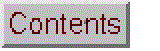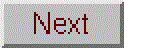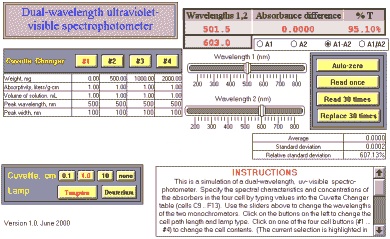Dual Wavelength U.V.-Vis. PhotometerVersion 1.0 (June, 2000). Click to see a larger view.

Simulation of a dual-wavelength uv-visible spectrophotometer with a 200 - 800 nm wavelength range, switchable tungsten and deuterium light sources, four interchangable quartz cuvettes, auto-zero button, four readout modes, and realistic sources of error and non-linearity. Students specify sample characteristics, select wavelength, cell path length, select deuterium or tungsten lamp, and perform measurements. Realistic modeling of lamp spectral characteristics, cell transmission variations, photon and detector noise, unabsorbed stray light, and instrument non-linearity caused by finite spectral bandpass.

Version 1.0 (June, 2000) has a fixed 5 nm spectral bandpass and models a single absorber in solution. Allows the student to specify the solute weight, solution volume, and the absorptivity, peak wavelength, and peak width of the absorber in each of the four cells. Version 1.1 (July, 2000) models a mixture of two absorbers (A and B) in solution.

Wingz player application and basic set of simulation modules, for windows PCs or Macintosh

Operating Instructions:

Specify the spectral characteristics and concentrations of the absorbers in the four cell by typing values into the Cuvette Changer table (cells C9 .. F13). Use the sliders above to change the wavelengths of the two monochromators. Click on the buttons on the lower left to change the cell path length and lamp type. Click on one of the four cuvette buttons (#1 ... #4) to change the cuvette in the light beam. (The current selections are highlighted in red). Click one of the four radio buttons below the absorbance readout to select the readout mode: A1 (absorbance at wavelength 1), A2 (absorbance at wavelength 2), difference mode (A1-A2), or ratio mode (A1/A2). Click the Auto-zero button to zero the readout on the current cell contents. Click the Read once button to take a single reading of absorbance. Click on the Read 30 times button to take 30 readings in quick succession (without removing and replacing the cell) and calculate the mean (average), standard deviation, and percent relative standard deviation of the readout. Click on the Replace 30 times button to simulate removing and replacing the cuvette 30 times (including the effect of changes in background transmission due to cell repositioning error, dust on the cell, particles in solution, etc).

Cell definitions and equations

Inputs:

Cuvette Changer table:
Weight, mg Cell #1 Cell #2 Cell #3 Cell #4
Absorptivity, liters/g-cm C9 D9 E9 F9
Volume of solution. mL C10 D10 E10 F10
Peak wavelength, nm C11 D11 E11 F11
Peak width, nm C12 D12 E12 F12

Wavelength1 (cell G4), controled by the slider
Wavelength2 (cell G5), controled by the slider
path (cell M3): absorption path length in cm, set by the three
"Cuvette, cm" buttons (0.1, 1, 10).
background (cell O3, mirrored in cell R6), default value 0.03
LampInt (cell M6), set by lamp buttons (see lamp button scripts below)
LampMax (cell M7), set by lamp buttons (see lamp button scripts below)
LampWidth (cell M8), set by lamp buttons (see lamp button scripts below)
Stray light = (cell S6), default value 0.01%
Photon noise factor (cell W6) default value 0.0001
Detector noise factor (cell X6), default value 0.0004

Calculated quantities

Theoretical peak absorbance table:
Cell # analyte absorbance
1 =0.001*C9*C10*path/C11
2 =0.001*D9*D10*path/D11
3 =0.001*E9*E10*path/E11
4 =0.001*F9*F10*path/F11

Spectral characteristics table:
M O R
Lamp Analyte Background
6 height 1.00 *** =background
7 position **** 500.00 150.00
8 width **** 100.00 1000.00

*** set by cell buttons
**** set by lamp buttons

Array calculations:

(Wavelength 1)
L9..L19: slice=wavelength1-5
M9..M19: Lamp=M\$6*exp(-((slice-M\$7)/M\$8)^2)
N9..N19: slit width = trangular function, 5 nm half-width
O9..O19: analyte=O\$6*exp(-((slice-O\$7)/O\$8)^2)
P9..P19: Interference=P\$6*exp(-((slice-P\$7)/P\$8)^2)
Q9..Q19: reagent=Q\$6*exp(-((slice-Q\$7)/Q\$8)^2)
R9..R19: background=R\$6*exp(-((slice-R\$7)/R\$8)^2)

T9..T19: Total absorbance = path*sum(\$O9..\$Q9)+\$R9
U9..U19: Incident intensity = \$M9+\$S\$6
V9..V19: Transmitted intensity = \$S\$6+\$M9*10^(-\$T9)
W9..W19: photon incident = \$W\$6*sqrt(\$V9)*rand()
X9..X19: detector incident = \$X\$6*rand()
Y9..Y19: photon trans = \$W\$6*sqrt(\$V9)*rand()
Z9..Z19: detector trans = \$X\$6*rand()
AA9..AA19: meas. I-zero = \$U9+\$W9+\$X9
AB9..AB19: Meas. I = \$V9+\$Y9+\$Z9

Outputs:
AA20: total meas I-zero = sum(\$AA9..\$AA19)
AB20: total meas I = sum(\$AB9..\$AB19)
AB21: absorbance = log(\$AA\$20/\$AB\$20)
I4: Absorbance (zero corrected) = \$AB\$21-\$G\$24
J4: %T = \$AB\$20/\$AA\$20

Statistics table
Average =avg(AC23..AC53)
Standard deviation =stdev(AC23..AC53)
Relative standard deviation =J17/J16

Button scripts:

"Cuvetter Changer" buttons:

#1
put 0.02+0.01*rand() into background

put C33 into O6
put C12 into O7
put C13 into O8
recalc

#2
put 0.02+0.01*rand() into background

put D33 into O6
put D12 into O7
put D13 into O8
recalc

and so on for cells 3 and 4.

"Cuvette, cm" buttons:

"0.1"
put 0.1 into path
put 0.02+0.01*rand() into background

recalc

and so forth for the 1.0 and 10 cm buttons.

"none"
put 0 into path
put 0 into background

recalc

Lamp buttons

Tungsten:
put 600 into LampMax
put 2 into LampInt
put 120 into LampWidth

recalc

Deuterium:
put 200 into LampMax
put .5 into LampInt
put 120 into LampWidth

recalc

Autozero:
recalc
put \$AB\$21 into g24